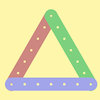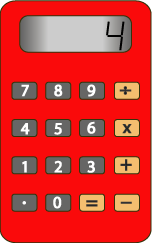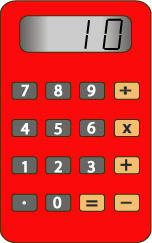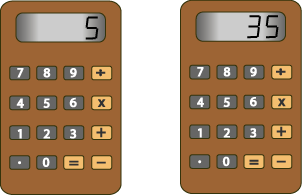#### You may also like### I'm Eight

Find a great variety of ways of asking questions which make 8.### Let's Investigate Triangles

Vincent and Tara are making triangles with the class construction set. They have a pile of strips of different lengths. How many different triangles can they make?### Noah

Noah saw 12 legs walk by into the Ark. How many creatures did he see?

# Secret Number

##### Age 5 to 7Challenge Level

This is a game for two players and a simple calculator.

Annie and Ben are playing. Annie puts her secret number into the calculator without showing Ben.

Annie then asks Ben, "What do you want to add?"

Ben tells Annie the number he wants to add. "I want to add four."

Annie presses the 'add' button and then the four button. The calculator now shows '$4$'. Annie gives the calculator to Ben.Ben presses the 'equals' button and the calculator gives the answer '$10$'.What was Annie's secret number?
How do you know?
You could play this with a friend. If you work out your friend's secret number correctly, it is your turn to put in a secret number of your own. You could score a point for every one you get right.

A multiplication version of the game might go like this:

Charlie puts in a secret number and asks Dana, "What do you want to multiply it by?"

Dana replies, "Multiply it by $5$."

Charlie puts in 'times' and '$5$' and hands the calculator to Dana.

When Dana presses the 'equals' button the calculator shows '$35$'.Dana now has to work out Charlie's secret number. What was it?
How do you know?

Try playing this version with your friend too!